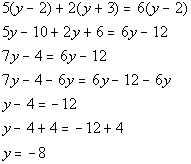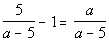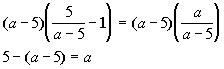TitleCollege Algebra
Answer/Discussion to Practice Problems
Tutorial 15: Equations with Rational Expressions

WTAMU > Virtual Math Lab > College Algebra > Tutorial 15: Equations with Rational ExpressionsAnswer/Discussion to 1aStep 1: Simplify by removing the fractions.*Factor 1st den.   *Mult. both sides by LCD of 2(y + 3)(y - 2)

 Step 2: Solve the remaining equation.*Remove ( ) by using dist. prop. *Get all y terms on one side   *Inverse of sub. 4 is add. 4

 Step 3: Check for extraneous solutions.

 Note that -8 does not cause any denominators to be zero.  So it is not an extraneous solution. -8 is the solution to our equation.Answer/Discussion to 1bStep 1: Simplify by removing the fractions.Step 2: Solve the remaining equation.*Remove ( ) by using dist. prop.   *Get all a terms on one side   *Inverse of add. 10 is sub. 10   *Inverse of mult. by -2 is div. by -2

 Step 3: Check for extraneous solutions.

 Note that 5 does cause two of the denominators to be zero.  So 5 is an extraneous solution.  That means there is no solution. The answer is NO solution.

WTAMU > Virtual Math Lab > College Algebra > Tutorial 15: Equations with Rational Expressions

Last revised on Dec. 16, 2009 by Kim Seward.
All contents copyright (C) 2002 - 2010, WTAMU and Kim Seward. All rights reserved.